# RD Sharma Solutions For Class 7 Maths Exercise 7.2 Chapter 7 Algebraic Expressions

Students can refer and download the PDF of RD Sharma Solutions for Class 7 Maths Exercise 7.2 of Chapter 7 Algebraic Expressions are available here. The questions present in this exercise has solved by the BYJU’S expert team. This exercise explains about operations on algebraic expressions. It includes 24 questions, along with many sub-questions. Some of the topics covered in this exercise are listed below:

• Addition of positive like terms
• Addition of negative like terms
• Addition of positive and negative like terms
• Addition of algebraic expressions with like and unlike terms
• Subtraction of algebraic expressions

It is suggested that students practice RD Sharma Solutions for Class 7 as many as time possible to understand the concepts easily.

## Download the PDF of RD Sharma Solutions For Class 7 Maths Chapter 7 – Algebraic Expressions Exercise 7.2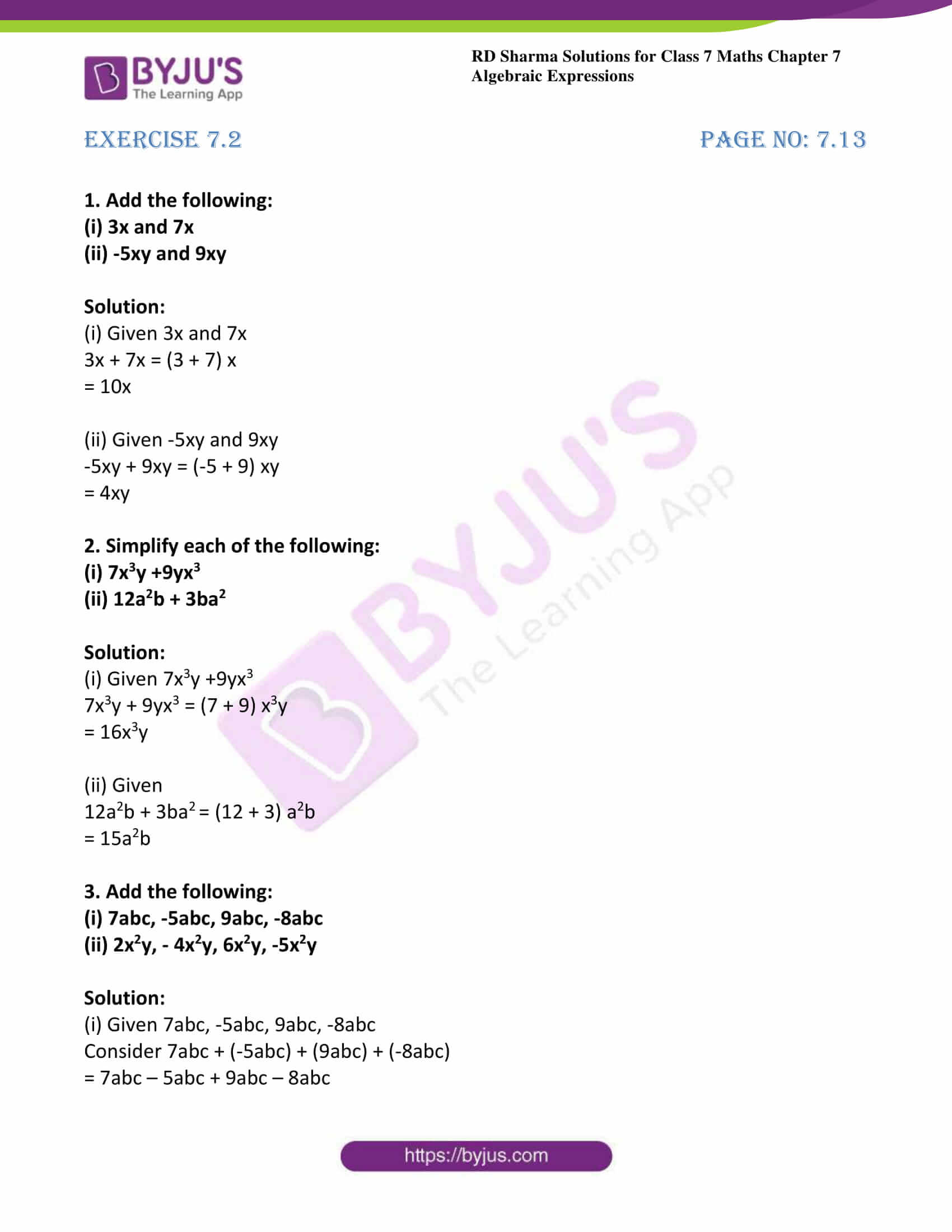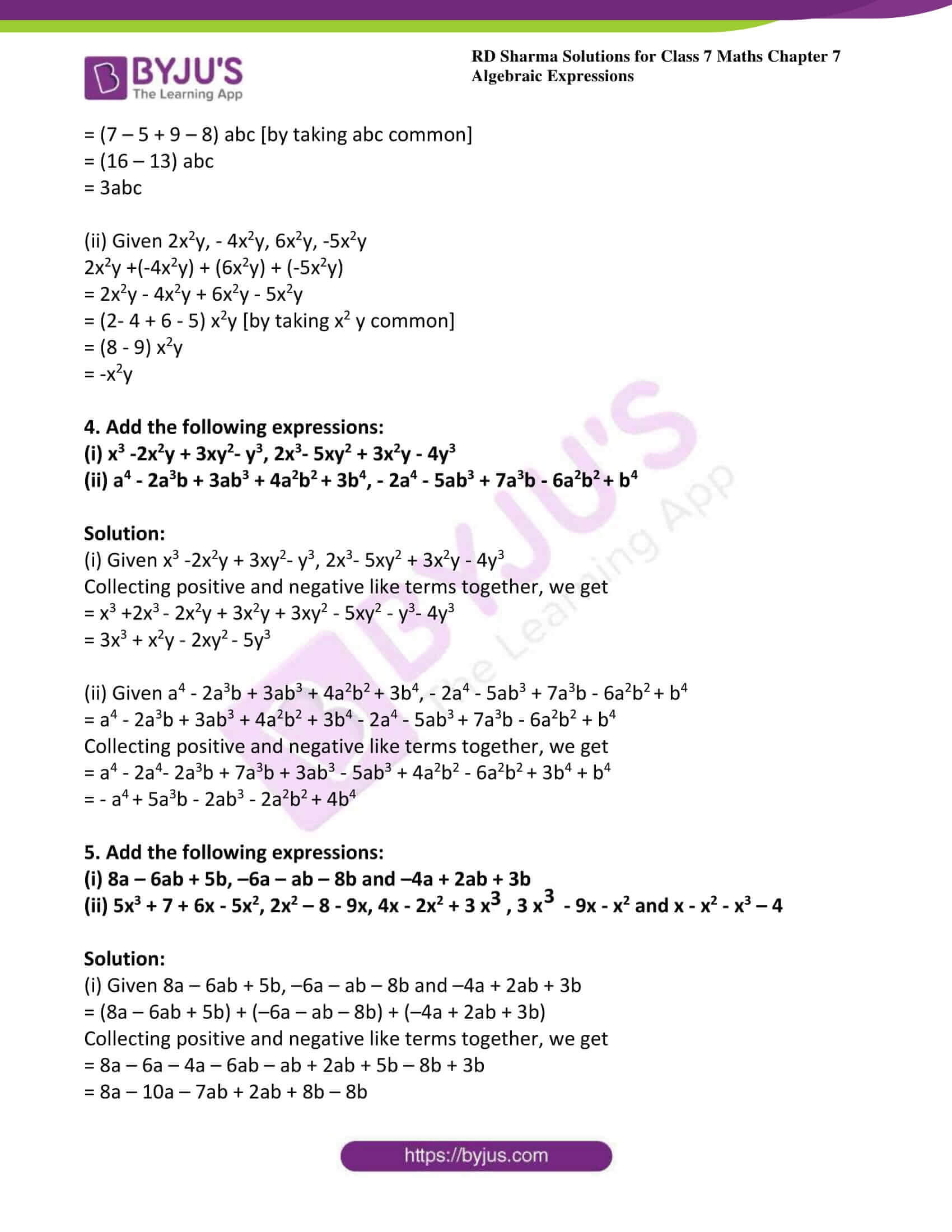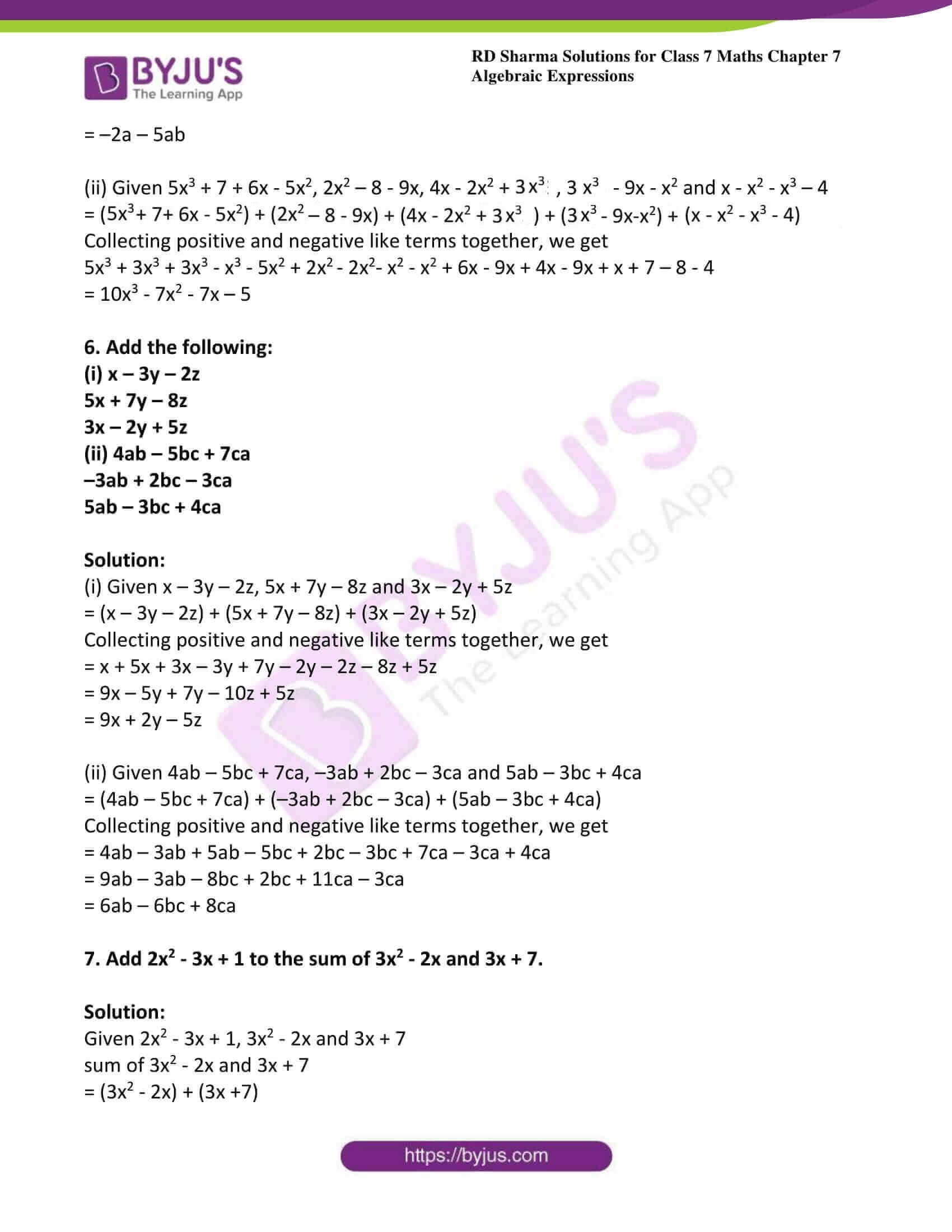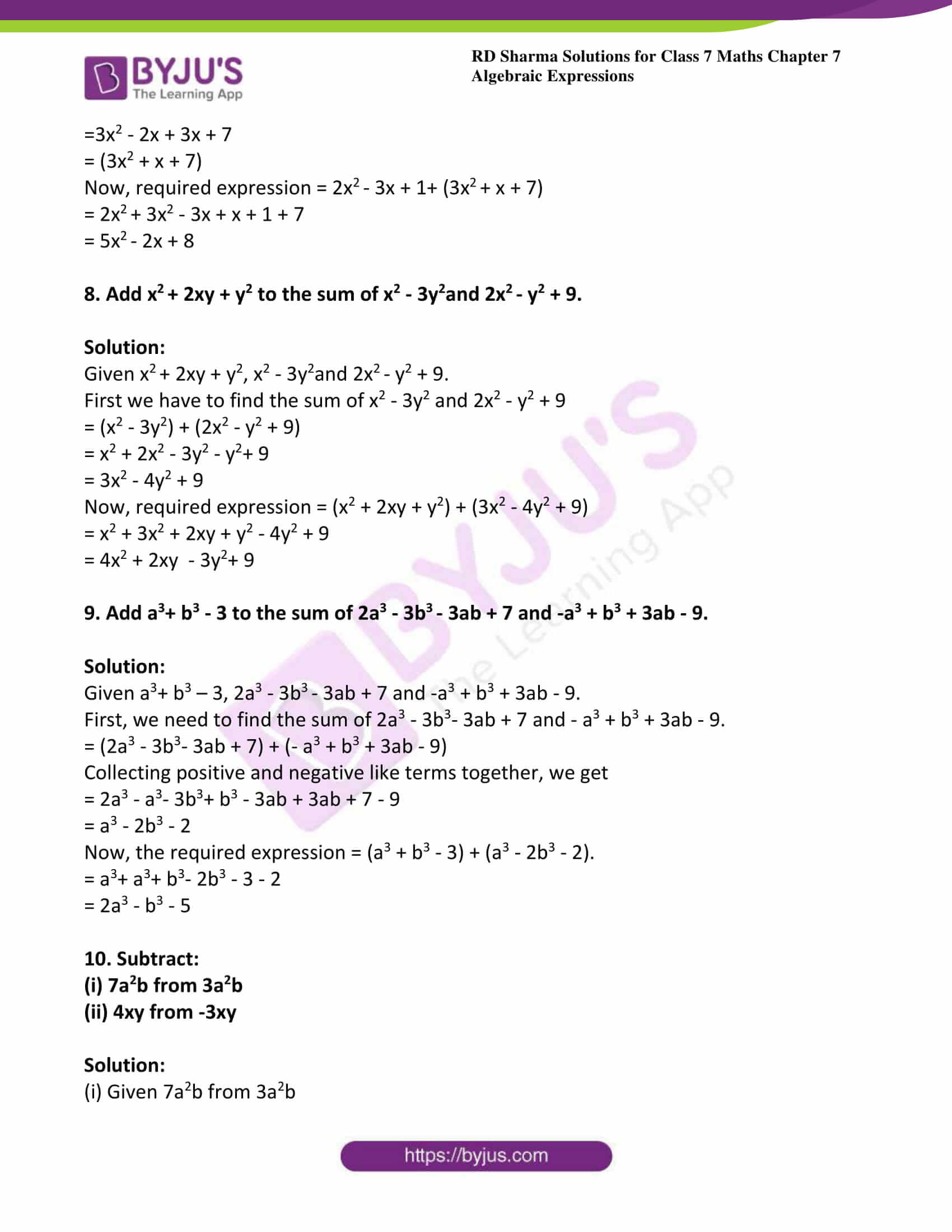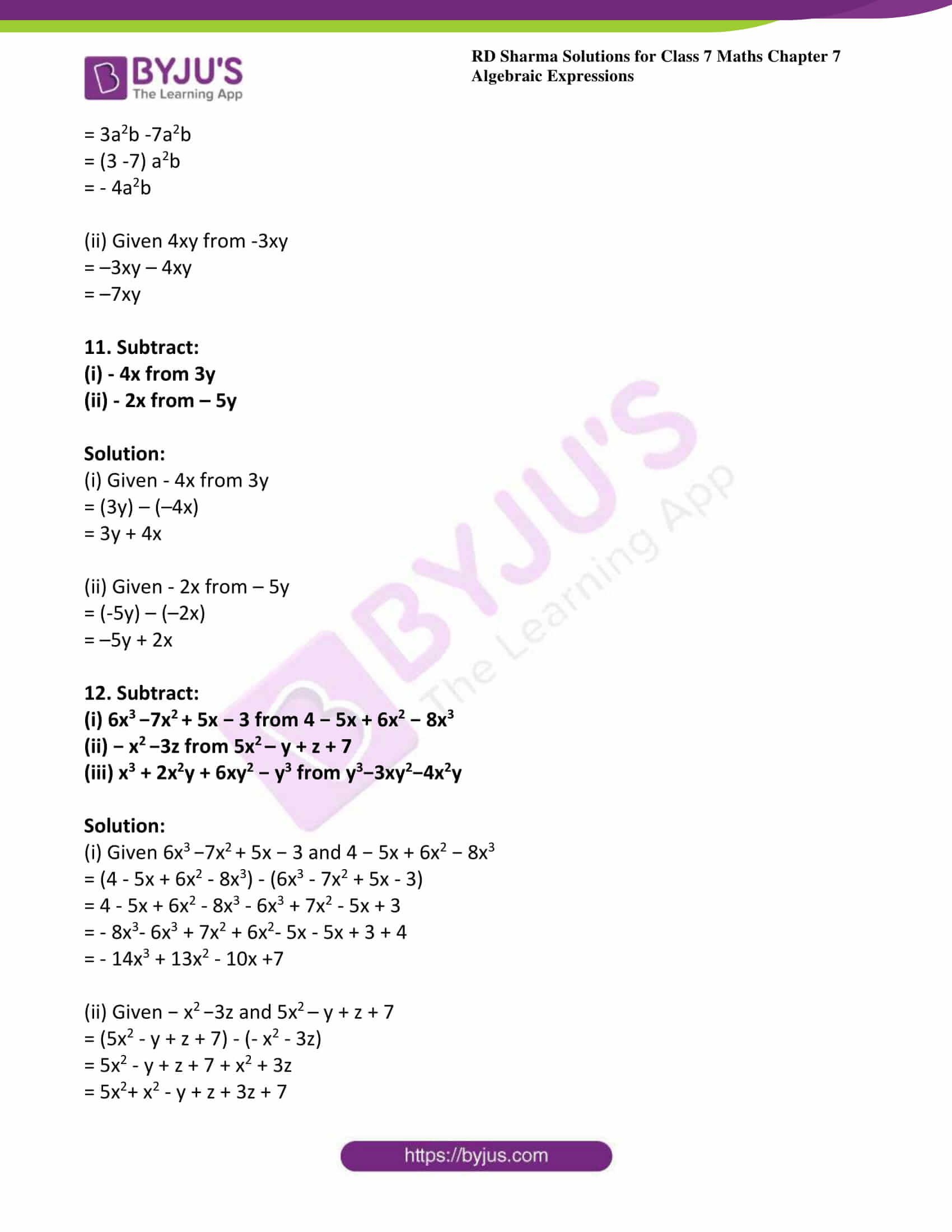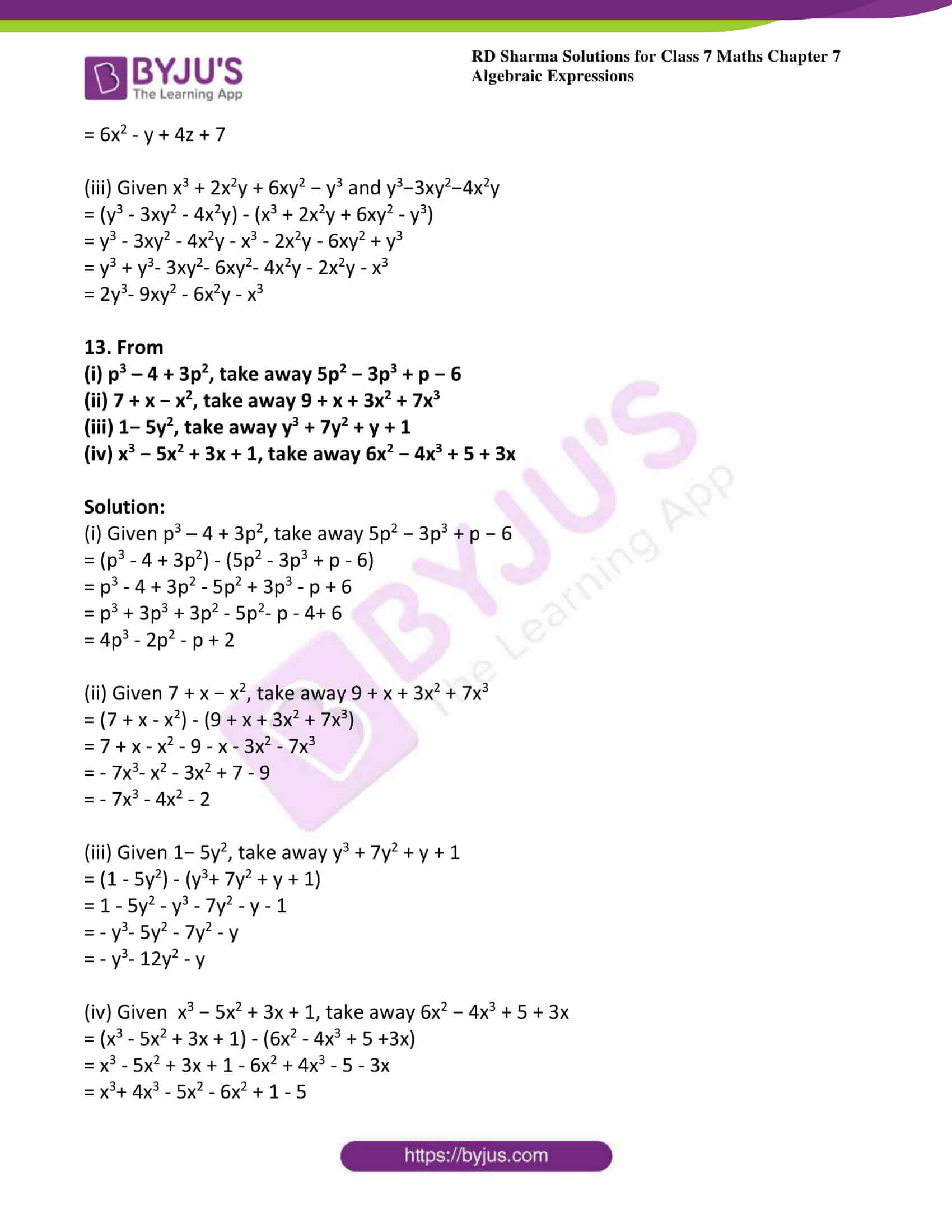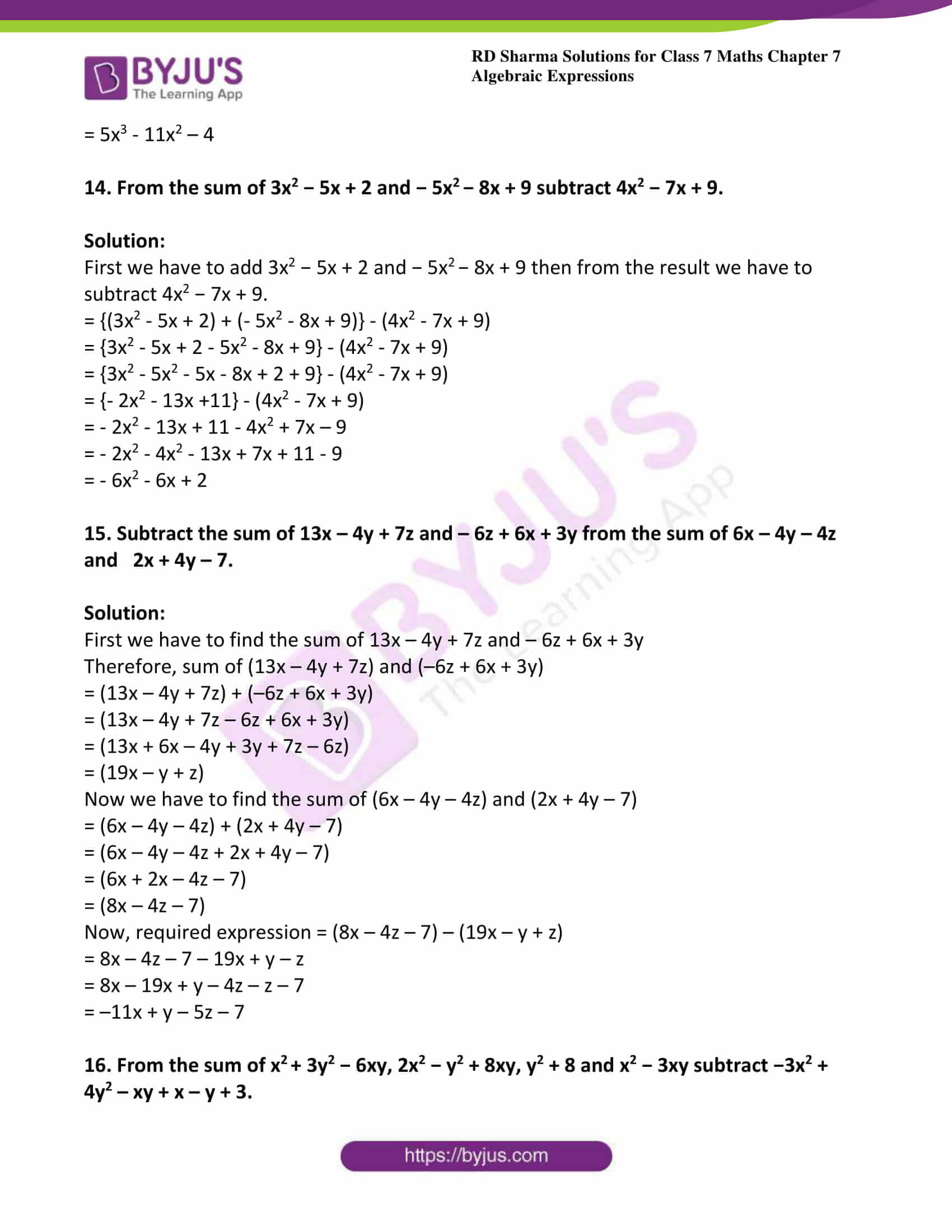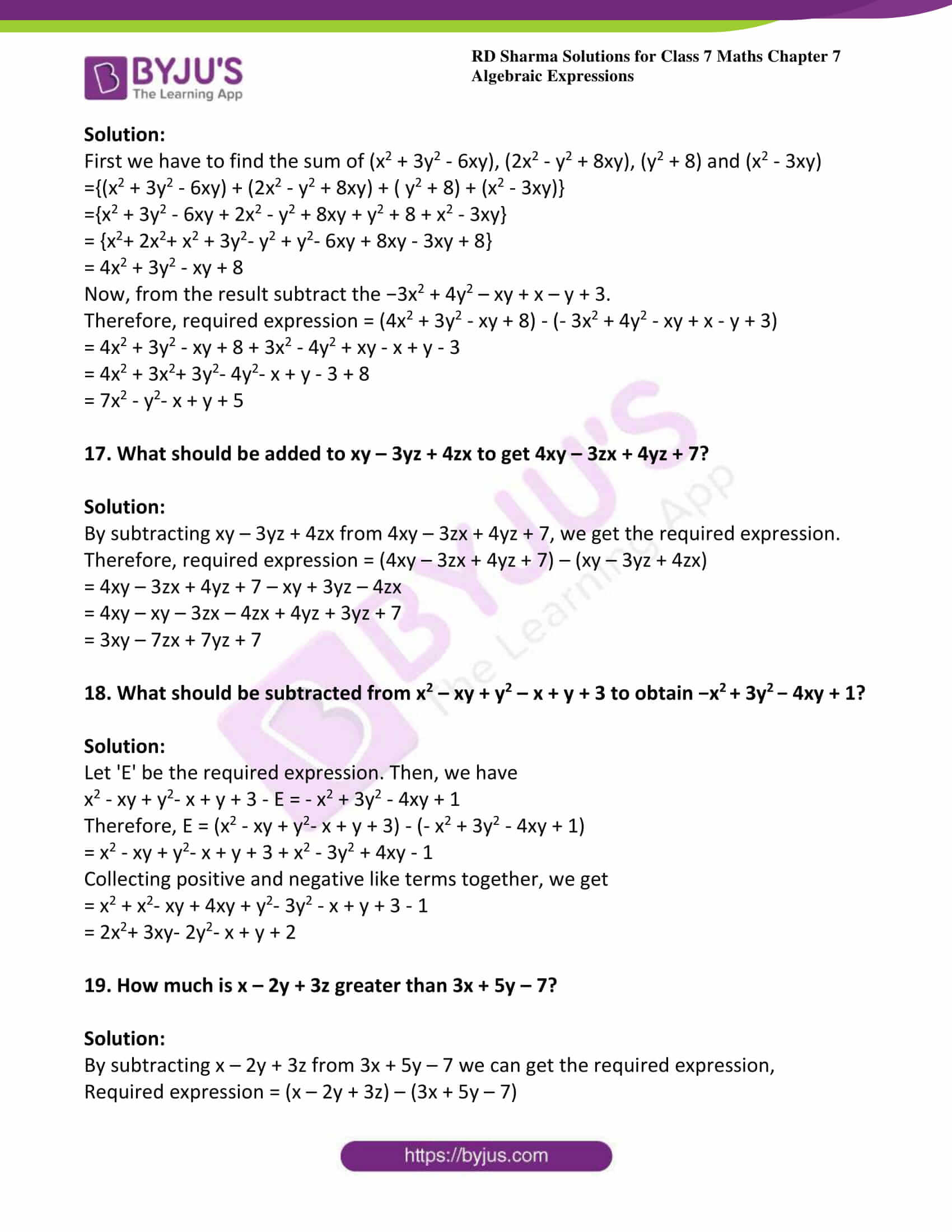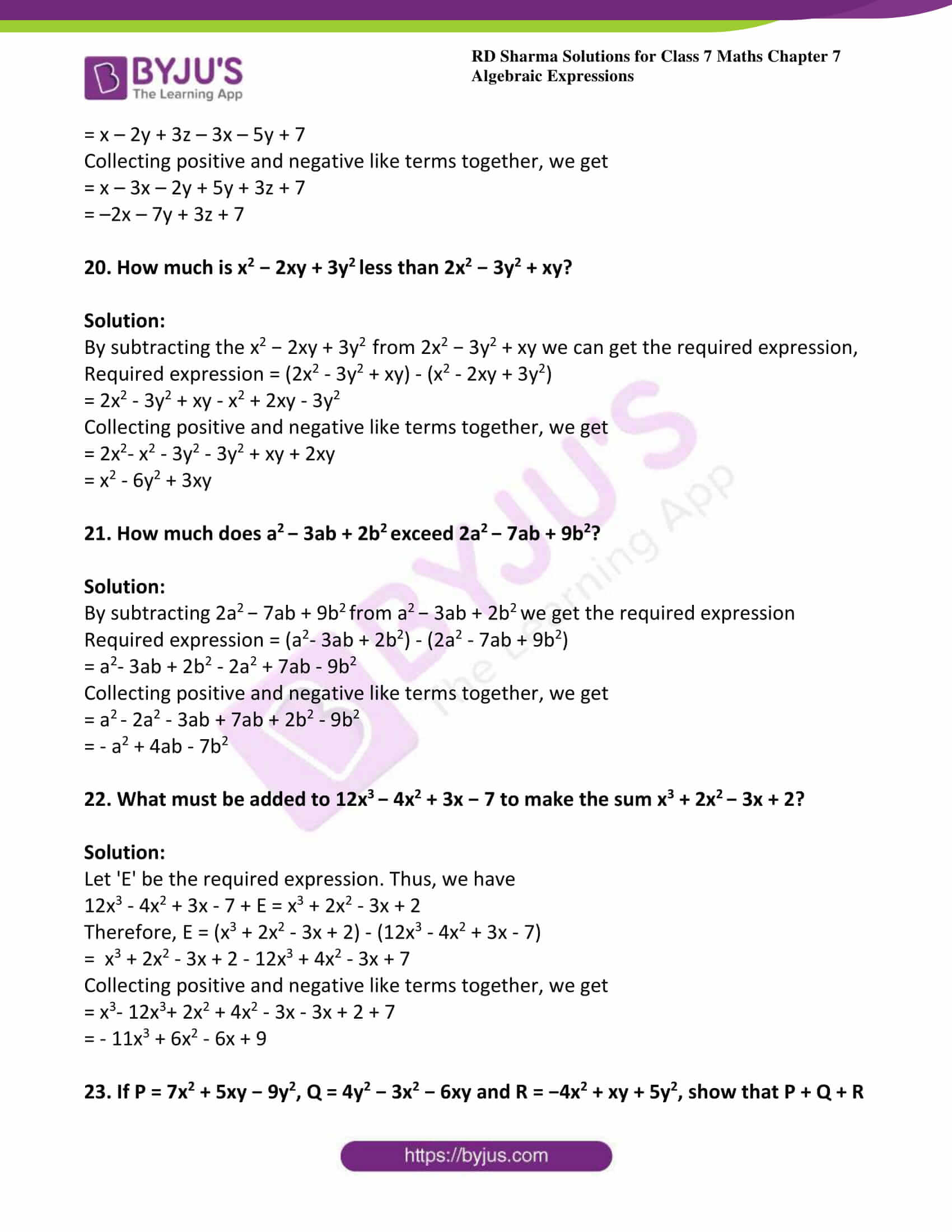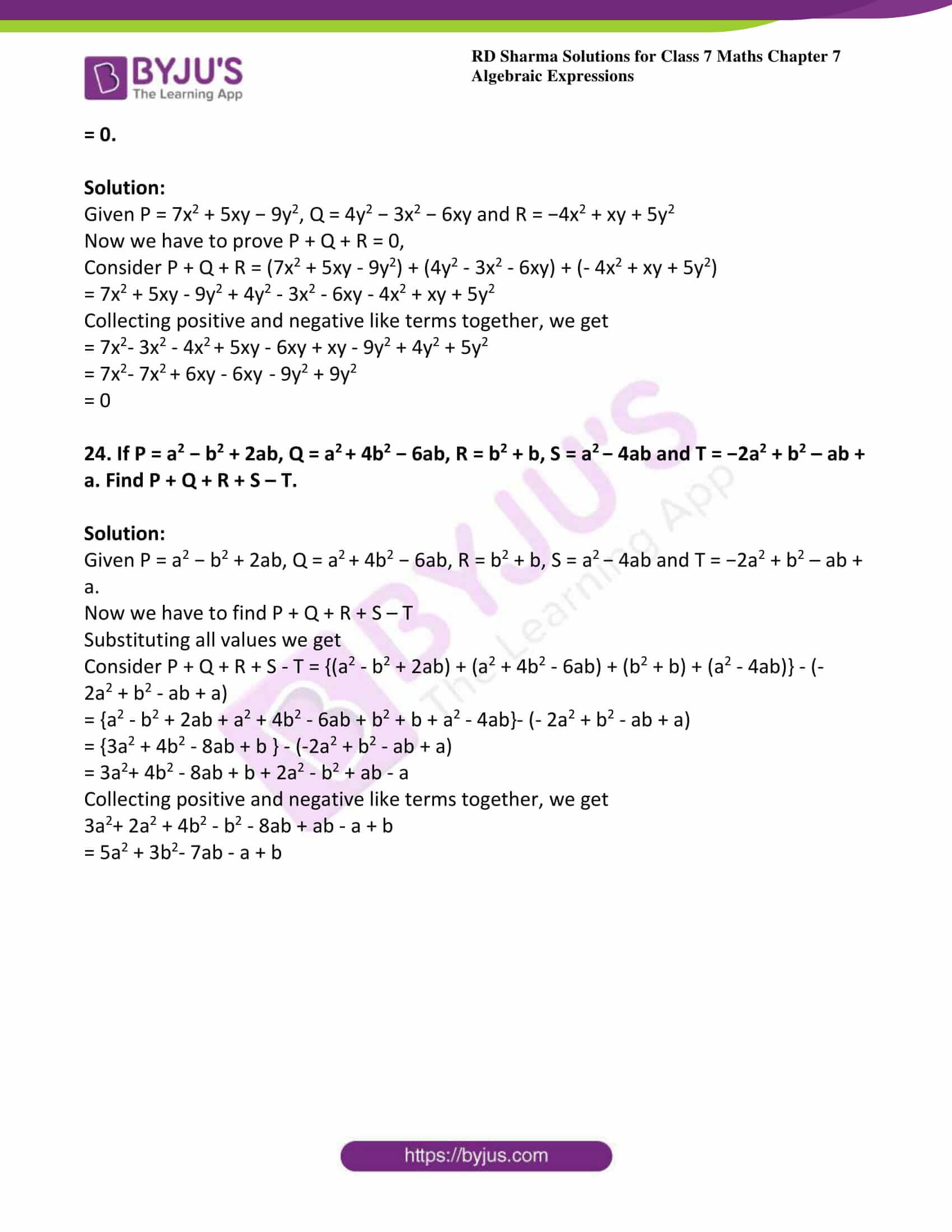### Access answers to Maths RD Sharma Solutions For Class 7 Chapter 7 – Algebraic Expressions Exercise 7.2

(i) 3x and 7x

(ii) -5xy and 9xy

Solution:

(i) Given 3x and 7x

3x + 7x = (3 + 7) x

= 10x

(ii) Given -5xy and 9xy

-5xy + 9xy = (-5 + 9) xy

= 4xy

2. Simplify each of the following:

(i) 7x3y +9yx3

(ii) 12a2b + 3ba2

Solution:

(i) Given 7x3y +9yx3

7x3y + 9yx3 = (7 + 9) x3y

= 16x3y

(ii) Given

12a2b + 3ba= (12 + 3) a2b

= 15a2b

(i) 7abc, -5abc, 9abc, -8abc

(ii) 2x2y, – 4x2y, 6x2y, -5x2y

Solution:

(i) Given 7abc, -5abc, 9abc, -8abc

Consider 7abc + (-5abc) + (9abc) + (-8abc)

= 7abc – 5abc + 9abc – 8abc

= (7 – 5 + 9 – 8) abc [by taking abc common]

= (16 – 13) abc

= 3abc

(ii) Given 2x2y, – 4x2y, 6x2y, -5x2y

2x2y +(-4x2y) + (6x2y) + (-5x2y)

= 2x2y – 4x2y + 6x2y – 5x2y

= (2- 4 + 6 – 5) x2y [by taking x2 y common]

= (8 – 9) x2y

= -x2y

(i) x3 -2x2y + 3xy2– y3, 2x3– 5xy2 + 3x2y – 4y3

(ii) a4 – 2a3b + 3ab3 + 4a2b+ 3b4, – 2a4 – 5ab3 + 7a3b – 6a2b+ b4

Solution:

(i) Given x3 -2x2y + 3xy2– y3, 2x3– 5xy2 + 3x2y – 4y3

Collecting positive and negative like terms together, we get

= x3 +2x– 2x2y + 3x2y + 3xy2 – 5xy2 – y3– 4y3

= 3x3 + x2y – 2xy– 5y3

(ii) Given a4 – 2a3b + 3ab3 + 4a2b+ 3b4, – 2a4 – 5ab3 + 7a3b – 6a2b+ b4

= a4 – 2a3b + 3ab3 + 4a2b2 + 3b4 – 2a4 – 5ab+ 7a3b – 6a2b2 + b4

Collecting positive and negative like terms together, we get

= a4 – 2a4– 2a3b + 7a3b + 3ab3 – 5ab3 + 4a2b2 – 6a2b+ 3b4 + b4

= – a+ 5a3b – 2ab3 – 2a2b+ 4b4

(i) 8a – 6ab + 5b, –6a – ab – 8b and –4a + 2ab + 3b

(ii) 5x3 + 7 + 6x – 5x2, 2x2 – 8 – 9x, 4x – 2x2 + 3 x 3, 3 x 3 – 9x – x2 and x – x2 – x3 – 4

Solution:

(i) Given 8a – 6ab + 5b, –6a – ab – 8b and –4a + 2ab + 3b

= (8a – 6ab + 5b) + (–6a – ab – 8b) + (–4a + 2ab + 3b)

Collecting positive and negative like terms together, we get

= 8a – 6a – 4a – 6ab – ab + 2ab + 5b – 8b + 3b

= 8a – 10a – 7ab + 2ab + 8b – 8b

= –2a – 5ab

(ii) Given 5x3 + 7 + 6x – 5x2, 2x2 – 8 – 9x, 4x – 2x2 + 3 x 3, 3 x 3 – 9x – x2 and x – x2 – x3 – 4

= (5 x 3 + 7+ 6x – 5x2) + (2 x2 – 8 – 9x) + (4x – 2x2 + 3 x 3) + (3 x3 – 9x-x2) + (x – x2 – x3 – 4)

Collecting positive and negative like terms together, we get

5x3 + 3x3 + 3x3 – x3 – 5x2 + 2x– 2x2– x2 – x2 + 6x – 9x + 4x – 9x + x + 7 – 8 – 4

= 10x3 – 7x2 – 7x – 5

(i) x – 3y – 2z

5x + 7y – 8z

3x – 2y + 5z

(ii) 4ab – 5bc + 7ca

–3ab + 2bc – 3ca

5ab – 3bc + 4ca

Solution:

(i) Given x – 3y – 2z, 5x + 7y – 8z and 3x – 2y + 5z

= (x – 3y – 2z) + (5x + 7y – 8z) + (3x – 2y + 5z)

Collecting positive and negative like terms together, we get

= x + 5x + 3x – 3y + 7y – 2y – 2z – 8z + 5z

= 9x – 5y + 7y – 10z + 5z

= 9x + 2y – 5z

(ii) Given 4ab – 5bc + 7ca, –3ab + 2bc – 3ca and 5ab – 3bc + 4ca

= (4ab – 5bc + 7ca) + (–3ab + 2bc – 3ca) + (5ab – 3bc + 4ca)

Collecting positive and negative like terms together, we get

= 4ab – 3ab + 5ab – 5bc + 2bc – 3bc + 7ca – 3ca + 4ca

= 9ab – 3ab – 8bc + 2bc + 11ca – 3ca

= 6ab – 6bc + 8ca

7. Add 2x2 – 3x + 1 to the sum of 3x2 – 2x and 3x + 7.

Solution:

Given 2x2 – 3x + 1, 3x2 – 2x and 3x + 7

sum of 3x2 – 2x and 3x + 7

= (3x2 – 2x) + (3x +7)

=3x2 – 2x + 3x + 7

= (3x2 + x + 7)

Now, required expression = 2x– 3x + 1+ (3x+ x + 7)

= 2x+ 3x2 – 3x + x + 1 + 7

= 5x– 2x + 8

8. Add x+ 2xy + y2 to the sum of x2 – 3y2and 2x– y2 + 9.

Solution:

Given x+ 2xy + y2, x2 – 3y2and 2x– y2 + 9.

First we have to find the sum of x2 – 3y2 and 2x2 – y2 + 9

= (x2 – 3y2) + (2x2 – y2 + 9)

= x2 + 2x2 – 3y2 – y2+ 9

= 3x2 – 4y2 + 9

Now, required expression = (x2 + 2xy + y2) + (3x2 – 4y2 + 9)

= x2 + 3x2 + 2xy + y2 – 4y2 + 9

= 4x2 + 2xy  – 3y2+ 9

9. Add a3+ b3 – 3 to the sum of 2a3 – 3b– 3ab + 7 and -a3 + b3 + 3ab – 9.

Solution:

Given a3+ b3 – 3, 2a3 – 3b– 3ab + 7 and -a3 + b3 + 3ab – 9.

First, we need to find the sum of 2a3 – 3b3– 3ab + 7 and – a3 + b3 + 3ab – 9.

= (2a3 – 3b3– 3ab + 7) + (- a3 + b3 + 3ab – 9)

Collecting positive and negative like terms together, we get

= 2a3 – a3– 3b3+ b3 – 3ab + 3ab + 7 – 9

= a3 – 2b3 – 2

Now, the required expression = (a3 + b3 – 3) + (a3 – 2b3 – 2).

= a3+ a3+ b3– 2b3 – 3 – 2

= 2a3 – b3 – 5

10. Subtract:

(i) 7a2b from 3a2b

(ii) 4xy from -3xy

Solution:

(i) Given 7a2b from 3a2b

= 3a2b -7a2b

= (3 -7) a2b

= – 4a2b

(ii) Given 4xy from -3xy

= –3xy – 4xy

= –7xy

11. Subtract:

(i) – 4x from 3y

(ii) – 2x from – 5y

Solution:

(i) Given – 4x from 3y

= (3y) – (–4x)

= 3y + 4x

(ii) Given – 2x from – 5y

= (-5y) – (–2x)

= –5y + 2x

12. Subtract:

(i) 6x−7x+ 5x − 3 from 4 − 5x + 6x2 − 8x3

(ii) − x−3z from 5x– y + z + 7

(iii) x3 + 2x2y + 6xy2 − y3 from y3−3xy2−4x2y

Solution:

(i) Given 6x−7x+ 5x − 3 and 4 − 5x + 6x2 − 8x3

= (4 – 5x + 6x2 – 8x3) – (6x3 – 7x2 + 5x – 3)

= 4 – 5x + 6x2 – 8x3 – 6x3 + 7x2 – 5x + 3

= – 8x3– 6x3 + 7x2 + 6x2– 5x – 5x + 3 + 4

= – 14x3 + 13x2 – 10x +7

(ii) Given − x−3z and 5x– y + z + 7

= (5x2 – y + z + 7) – (- x2 – 3z)

= 5x2 – y + z + 7 + x2 + 3z

= 5x2+ x2 – y + z + 3z + 7

= 6x2 – y + 4z + 7

(iii) Given x3 + 2x2y + 6xy2 − y3 and y3−3xy2−4x2y

= (y3 – 3xy2 – 4x2y) – (x3 + 2x2y + 6xy2 – y3)

= y3 – 3xy2 – 4x2y – x3 – 2x2y – 6xy2 + y3

= y3 + y3– 3xy2– 6xy2– 4x2y – 2x2y – x3

= 2y3– 9xy2 – 6x2y – x3

13. From

(i) p3 – 4 + 3p2, take away 5p2 − 3p3 + p − 6

(ii) 7 + x − x2, take away 9 + x + 3x2 + 7x3

(iii) 1− 5y2, take away y3 + 7y2 + y + 1

(iv) x3 − 5x2 + 3x + 1, take away 6x2 − 4x3 + 5 + 3x

Solution:

(i) Given p3 – 4 + 3p2, take away 5p2 − 3p3 + p − 6

= (p3 – 4 + 3p2) – (5p2 – 3p3 + p – 6)

= p3 – 4 + 3p2 – 5p2 + 3p3 – p + 6

= p3 + 3p3 + 3p2 – 5p2– p – 4+ 6

= 4p3 – 2p2 – p + 2

(ii) Given 7 + x − x2, take away 9 + x + 3x2 + 7x3

= (7 + x – x2) – (9 + x + 3x2 + 7x3)

= 7 + x – x2 – 9 – x – 3x2 – 7x3

= – 7x3– x2 – 3x2 + 7 – 9

= – 7x3 – 4x2 – 2

(iii) Given 1− 5y2, take away y3 + 7y2 + y + 1

= (1 – 5y2) – (y3+ 7y2 + y + 1)

= 1 – 5y2 – y3 – 7y2 – y – 1

= – y3– 5y2 – 7y2 – y

= – y3– 12y2 – y

(iv) Given x3 − 5x2 + 3x + 1, take away 6x2 − 4x3 + 5 + 3x

= (x3 – 5x2 + 3x + 1) – (6x2 – 4x3 + 5 +3x)

= x3 – 5x2 + 3x + 1 – 6x2 + 4x3 – 5 – 3x

= x3+ 4x3 – 5x2 – 6x2 + 1 – 5

= 5x3 – 11x2 – 4

14. From the sum of 3x2 − 5x + 2 and − 5x− 8x + 9 subtract 4x2 − 7x + 9.

Solution:

First we have to add 3x2 − 5x + 2 and − 5x− 8x + 9 then from the result we have to subtract 4x2 − 7x + 9.

= {(3x2 – 5x + 2) + (- 5x2 – 8x + 9)} – (4x2 – 7x + 9)

= {3x2 – 5x + 2 – 5x2 – 8x + 9} – (4x2 – 7x + 9)

= {3x2 – 5x2 – 5x – 8x + 2 + 9} – (4x2 – 7x + 9)

= {- 2x2 – 13x +11} – (4x2 – 7x + 9)

= – 2x2 – 13x + 11 – 4x2 + 7x – 9

= – 2x2 – 4x2 – 13x + 7x + 11 – 9

= – 6x2 – 6x + 2

15. Subtract the sum of 13x – 4y + 7z and – 6z + 6x + 3y from the sum of 6x – 4y – 4z and   2x + 4y – 7.

Solution:

First we have to find the sum of 13x – 4y + 7z and – 6z + 6x + 3y

Therefore, sum of (13x – 4y + 7z) and (–6z + 6x + 3y)

= (13x – 4y + 7z) + (–6z + 6x + 3y)

= (13x – 4y + 7z – 6z + 6x + 3y)

= (13x + 6x – 4y + 3y + 7z – 6z)

= (19x – y + z)

Now we have to find the sum of (6x – 4y – 4z) and (2x + 4y – 7)

= (6x – 4y – 4z) + (2x + 4y – 7)

= (6x – 4y – 4z + 2x + 4y – 7)

= (6x + 2x – 4z – 7)

= (8x – 4z – 7)

Now, required expression = (8x – 4z – 7) – (19x – y + z)

= 8x – 4z – 7 – 19x + y – z

= 8x – 19x + y – 4z – z – 7

= –11x + y – 5z – 7

16. From the sum of x+ 3y2 − 6xy, 2x2 − y2 + 8xy, y2 + 8 and x2 − 3xy subtract −3x2 + 4y2 – xy + x – y + 3.

Solution:

First we have to find the sum of (x2 + 3y2 – 6xy), (2x2 – y2 + 8xy), (y2 + 8) and (x2 – 3xy)

={(x2 + 3y2 – 6xy) + (2x2 – y2 + 8xy) + ( y2 + 8) + (x2 – 3xy)}

={x2 + 3y2 – 6xy + 2x2 – y2 + 8xy + y2 + 8 + x2 – 3xy}

= {x2+ 2x2+ x2 + 3y2– y2 + y2– 6xy + 8xy – 3xy + 8}

= 4x2 + 3y2 – xy + 8

Now, from the result subtract the −3x2 + 4y2 – xy + x – y + 3.

Therefore, required expression = (4x2 + 3y2 – xy + 8) – (- 3x2 + 4y2 – xy + x – y + 3)

= 4x2 + 3y2 – xy + 8 + 3x2 – 4y2 + xy – x + y – 3

= 4x2 + 3x2+ 3y2– 4y2– x + y – 3 + 8

= 7x2 – y2– x + y + 5

17. What should be added to xy – 3yz + 4zx to get 4xy – 3zx + 4yz + 7?

Solution:

By subtracting xy – 3yz + 4zx from 4xy – 3zx + 4yz + 7, we get the required expression.

Therefore, required expression = (4xy – 3zx + 4yz + 7) – (xy – 3yz + 4zx)

= 4xy – 3zx + 4yz + 7 – xy + 3yz – 4zx

= 4xy – xy – 3zx – 4zx + 4yz + 3yz + 7

= 3xy – 7zx + 7yz + 7

18. What should be subtracted from x2 – xy + y2 – x + y + 3 to obtain −x+ 3y− 4xy + 1?

Solution:

Let ‘E’ be the required expression. Then, we have

x2 – xy + y2– x + y + 3 – E = – x2 + 3y2 – 4xy + 1

Therefore, E = (x2 – xy + y2– x + y + 3) – (- x2 + 3y2 – 4xy + 1)

= x2 – xy + y2– x + y + 3 + x2 – 3y2 + 4xy – 1

Collecting positive and negative like terms together, we get

= x2 + x2– xy + 4xy + y2– 3y2 – x + y + 3 – 1

= 2x2+ 3xy- 2y2– x + y + 2

19. How much is x – 2y + 3z greater than 3x + 5y – 7?

Solution:

By subtracting x – 2y + 3z from 3x + 5y – 7 we can get the required expression,

Required expression = (x – 2y + 3z) – (3x + 5y – 7)

= x – 2y + 3z – 3x – 5y + 7

Collecting positive and negative like terms together, we get

= x – 3x – 2y + 5y + 3z + 7

= –2x – 7y + 3z + 7

20. How much is x2 − 2xy + 3yless than 2x2 − 3y2 + xy?

Solution:

By subtracting the x2 − 2xy + 3yfrom 2x2 − 3y2 + xy we can get the required expression,

Required expression = (2x2 – 3y2 + xy) – (x2 – 2xy + 3y2)

= 2x2 – 3y2 + xy – x2 + 2xy – 3y2

Collecting positive and negative like terms together, we get

= 2x2– x2 – 3y2 – 3y2 + xy + 2xy

= x2 – 6y2 + 3xy

21. How much does a− 3ab + 2bexceed 2a− 7ab + 9b2?

Solution:

By subtracting 2a− 7ab + 9b2 from a− 3ab + 2bwe get the required expression

Required expression = (a2– 3ab + 2b2) – (2a2 – 7ab + 9b2)

= a2– 3ab + 2b2 – 2a2 + 7ab – 9b2

Collecting positive and negative like terms together, we get

= a– 2a2 – 3ab + 7ab + 2b2 – 9b2

= – a2 + 4ab – 7b2

22. What must be added to 12x− 4x2 + 3x − 7 to make the sum x3 + 2x− 3x + 2?

Solution:

Let ‘E’ be the required expression. Thus, we have

12x3 – 4x2 + 3x – 7 + E = x3 + 2x2 – 3x + 2

Therefore, E = (x3 + 2x2 – 3x + 2) – (12x3 – 4x2 + 3x – 7)

=  x3 + 2x2 – 3x + 2 – 12x3 + 4x2 – 3x + 7

Collecting positive and negative like terms together, we get

= x3– 12x3+ 2x2 + 4x2 – 3x – 3x + 2 + 7

= – 11x3 + 6x2 – 6x + 9

23. If P = 7x2 + 5xy − 9y2, Q = 4y2 − 3x2 − 6xy and R = −4x2 + xy + 5y2, show that P + Q + R = 0.

Solution:

Given P = 7x2 + 5xy − 9y2, Q = 4y2 − 3x2 − 6xy and R = −4x2 + xy + 5y2

Now we have to prove P + Q + R = 0,

Consider P + Q + R = (7x2 + 5xy – 9y2) + (4y2 – 3x2 – 6xy) + (- 4x2 + xy + 5y2)

= 7x2 + 5xy – 9y2 + 4y2 – 3x2 – 6xy – 4x2 + xy + 5y2

Collecting positive and negative like terms together, we get

= 7x2– 3x2 – 4x+ 5xy – 6xy + xy – 9y2 + 4y2 + 5y2

= 7x2– 7x+ 6xy – 6xy  – 9y2 + 9y2

= 0

24. If P = a2 − b2 + 2ab, Q = a+ 4b2 − 6ab, R = b2 + b, S = a− 4ab and T = −2a2 + b2 – ab + a. Find P + Q + R + S – T.

Solution:

Given P = a2 − b2 + 2ab, Q = a+ 4b2 − 6ab, R = b2 + b, S = a− 4ab and T = −2a2 + b2 – ab + a.

Now we have to find P + Q + R + S – T

Substituting all values we get

Consider P + Q + R + S – T = {(a2 – b2 + 2ab) + (a2 + 4b2 – 6ab) + (b2 + b) + (a2 – 4ab)} – (-2a2 + b2 – ab + a)

= {a2 – b2 + 2ab + a2 + 4b2 – 6ab + b2 + b + a2 – 4ab}- (- 2a2 + b2 – ab + a)

= {3a2 + 4b2 – 8ab + b } – (-2a2 + b2 – ab + a)

= 3a2+ 4b2 – 8ab + b + 2a2 – b2 + ab – a

Collecting positive and negative like terms together, we get

3a2+ 2a2 + 4b2 – b2 – 8ab + ab – a + b

= 5a2 + 3b2– 7ab – a + b